Sine and Cosine Formula

Chapter 3 Class 11 Trigonometric Functions
Concept wise

For triangle Δ ABC

Sine Law is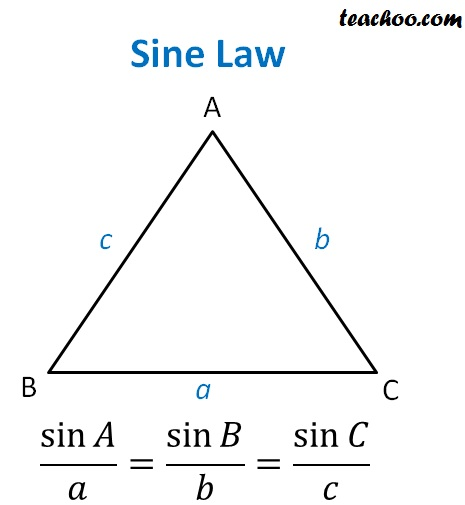Here

• A, B, C are vertices of Δ ABC
• a is side opposite to A i.e. BC
• b is side opposite to B i.e. AC
• c is side opposite to C i.e. AB

Let’s look at its proof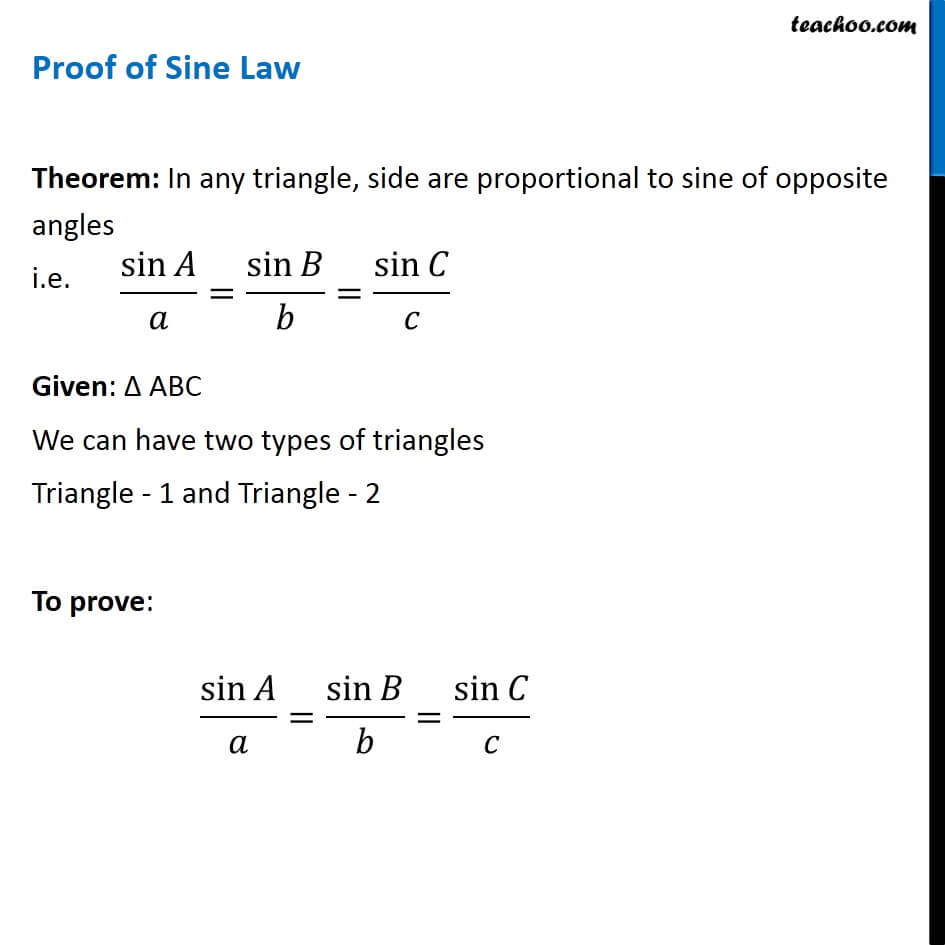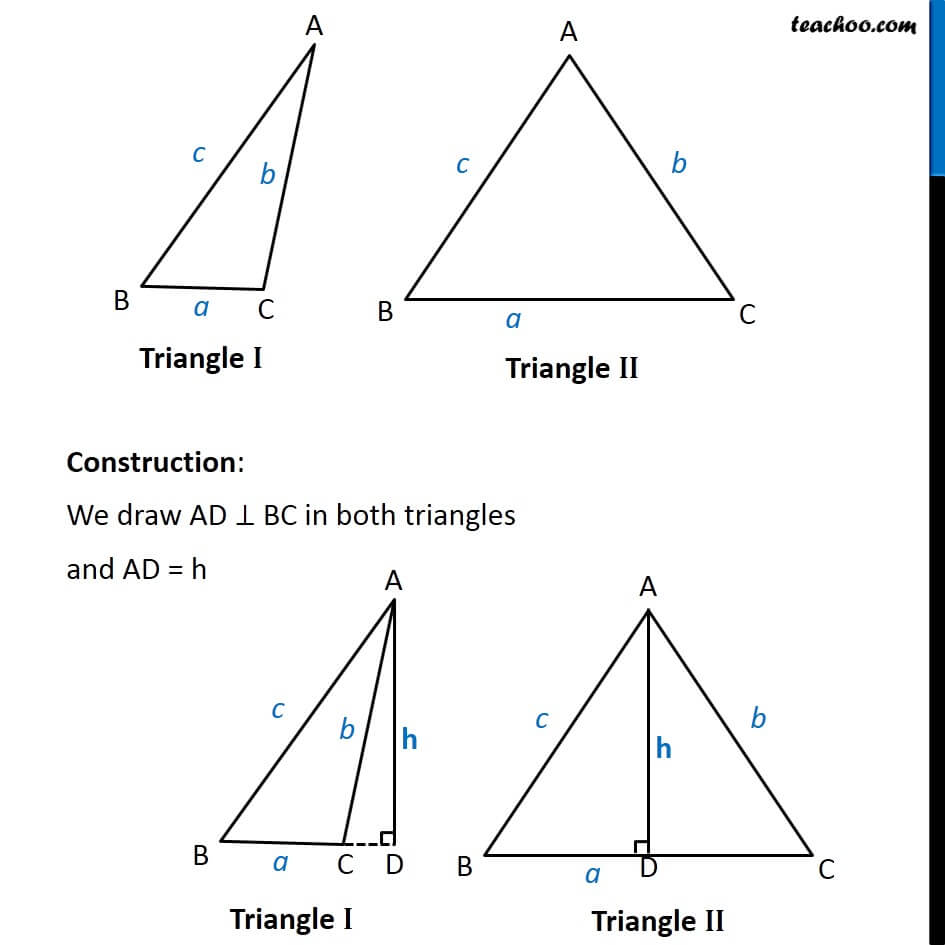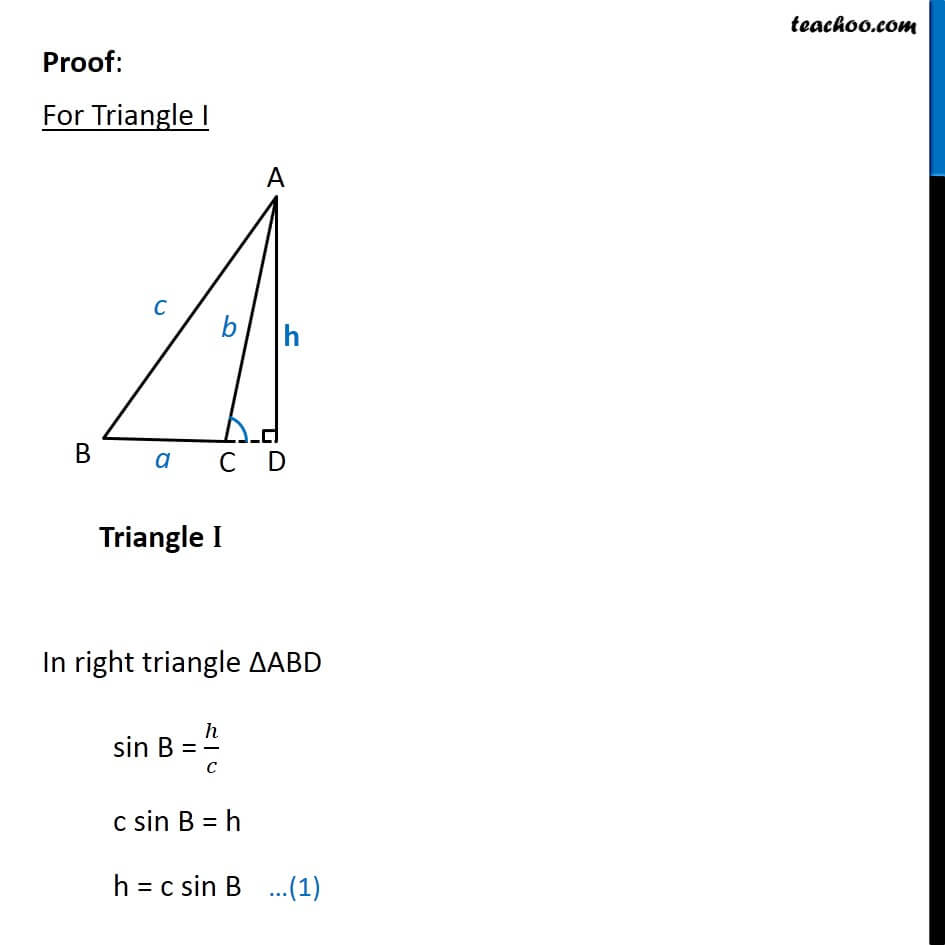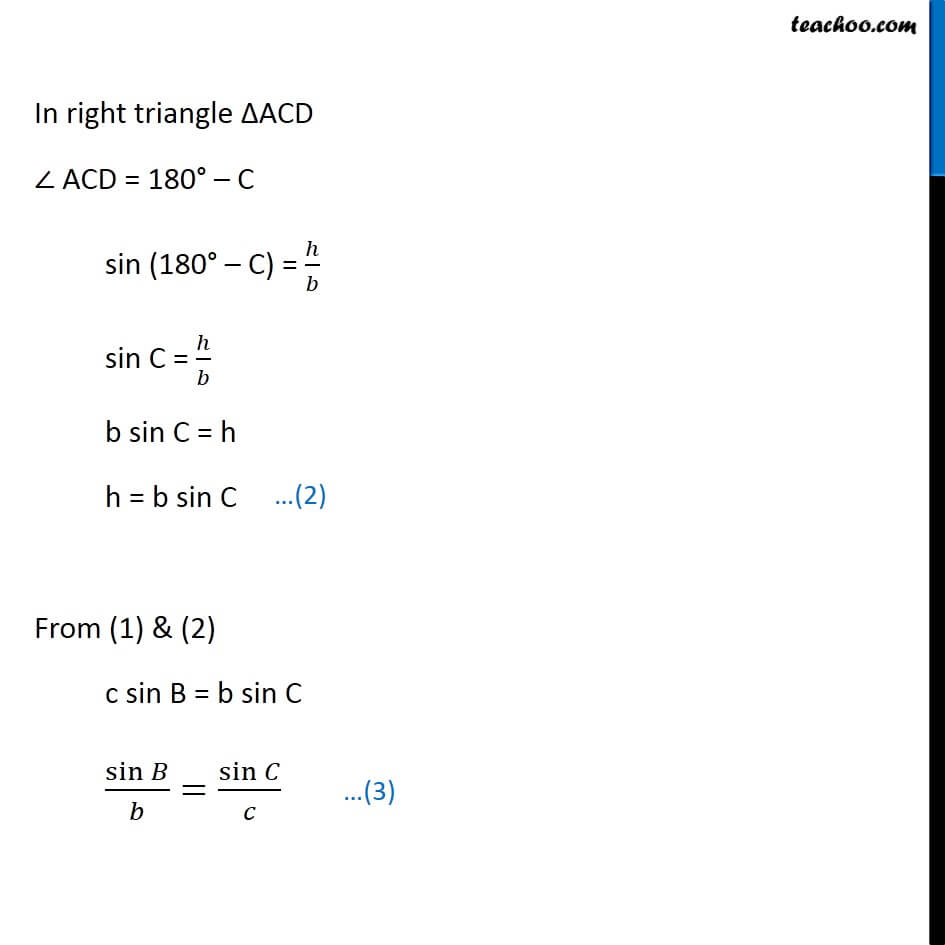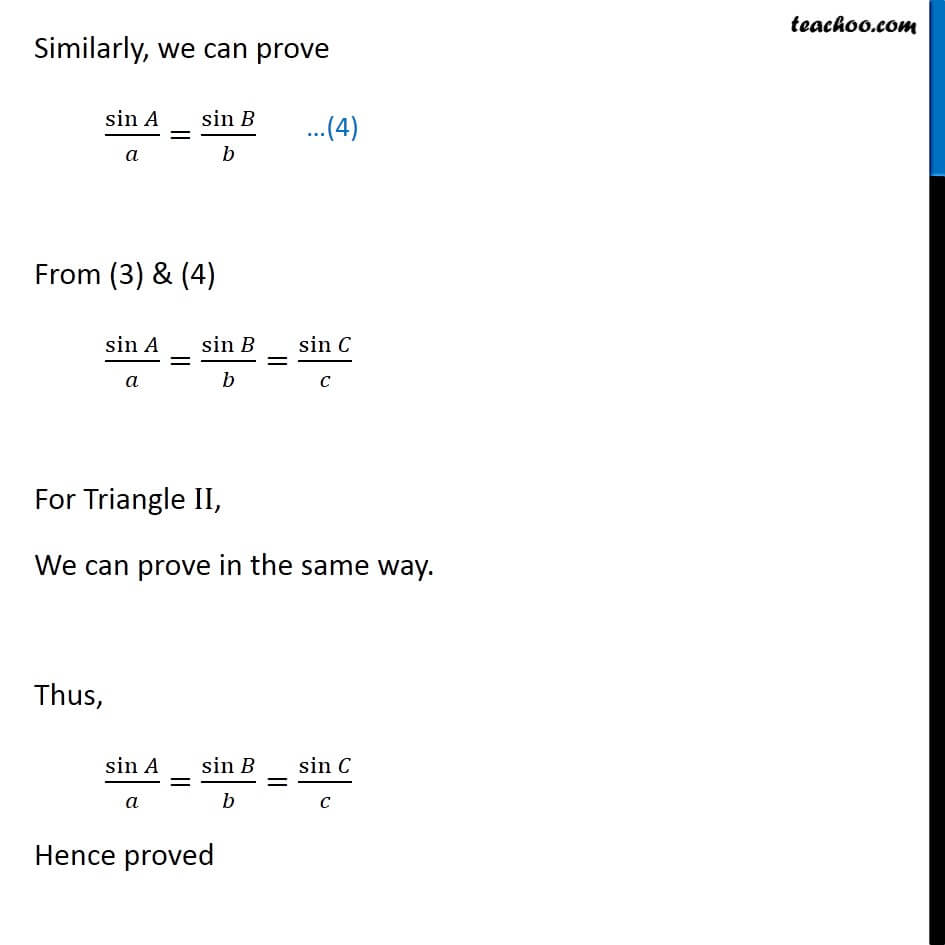Learn in your speed, with individual attention - Teachoo Maths 1-on-1 Class

### Transcript

For triangle ABC Sine Law is Here A, B, C are vertices of ABC a is side opposite to A i.e. BC b is side opposite to B i.e. AC c is side opposite to C i.e. AB Let s look at its proof